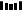# 数组特性的妙用！如何找到「缺失的第一个正数」### 题目描述

``输入: [1,2,0]输出: 3``

``输入: [3,4,-1,1]输出: 2``

``输入: [7,8,9,11,12]输出: 1``

### 题目解析

1. 缺失的正整数肯定在 [1, array.length + 1] 这个范围内

2. 我们可以交换输入数组中的元素的位置来让 index 和 value 的关系更加明确

3. 保证 index 和 value 的关系后，我们可以通过 index 来判定整数的存在性

### 代码实现

``public int firstMissingPositive(int[] nums) {    if (nums == null || nums.length == 0) {        return 1;    }    for (int i = 0; i < nums.length;) {        if (nums[i] <= 0 || nums[i] > nums.length || nums[nums[i] - 1] == nums[i]) {            i++;            continue;        }        // swap        int tmp = nums[nums[i] - 1];        nums[nums[i] - 1] = nums[i];        nums[i] = tmp;    }    for (int i = 0; i < nums.length; ++i) {        if (nums[i] != i + 1) {            return i + 1;        }    }    return nums.length + 1;}``

### 总结

• 第一点，交换完后，需要判断交换过来的数是否需要被放到相应的地方，例如 `[2,3,1]`

• 第二点，元素越界的话，以及元素 value 已经和 index 对应上了，那么就应该继续遍历，例如 `[0,-1,1]``[1,1]`1.程序员

2.【GitHub

3.【算法动画：七分钟理解什么是KMP算法

4.【数据结构十大经典排序算法动画与解析，看我就够了！